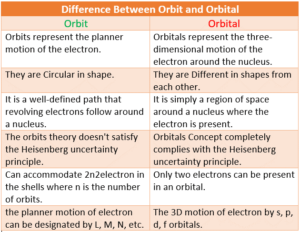# Quantum Mechanics and Quantum Numbers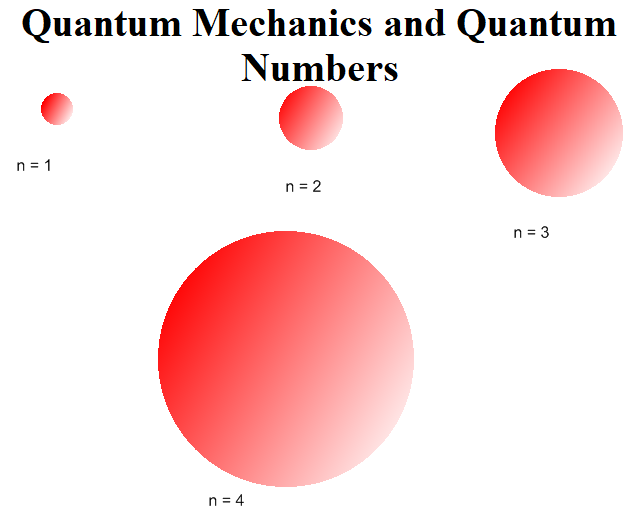Quantum Mechanics and Quantum Numbers

Quantum Mechanics and Quantum Numbers post provides complete information about quantum numbers with examples and wave function

Quantum Mechanics: Quantum mechanics takes into account the dual behavior of matter. An equation, given by Schrodinger, which has a better physical interpretation in terms of wave properties is Ĥ ψ = Eψ. Where Ĥ is called Hamiltonian operator, E is the total energy of the system (K.E + P.E) and ψ is called the wave function.

Physical Significance of ψ: The wave function (ψ) is a mathematical function and it has no physical significance. Wave functions of hydrogen or hydrogen-like species with one electron are called atomic orbitals. All the information about the electron in an atom is stored in its orbital wave function ψ.Its square ψ2 is proportional to the probability of finding the electron at a given point around the nucleus and is always positive.

Quantum numbers: There are a set of four quantum numbers that specify the energy, size, shape, and orientation of an orbital. The Quantum number gives the address of the electron.                                                                                                                                                        These are:
(i) Principal quantum number (n)
(ii) Azimuthal quantum number (l)
(iii) Magnetic quantum number (m)
(iv) Electron spin quantum number (ms)

Principal Quantum Number: The following formations are obtained from n.
1. It gives the size of the orbit.
2. It gives the energy of an electron in an orbit.
3. It gives the shell in which the electron is found.
4. It also gives the average distance between the electron and the nucleus. As the value of n increases, the distance between the electron and the nucleus also increases.

 n 1 2 3 4 Shell no. K L M N Total number of orbitals in a shell = n2 1 4 9 16 Maximum number of electrons = 2n2 2 8 18 32

Azimuthal quantum number (l): Azimuthal quantum number. ‘l’ is also known as orbital angular momentum or subsidiary quantum number. It identified the subshell and the three-dimensional shape of the orbital. It also determines the number of subshells or sub levels in a shell. The total number of subshells in a particular shell is equal to the value of n. l = 0, 1, 2… (n-1)
For example, when n = 1, the value of Ɩ is only 0.
For n = 2, the possible value of Ɩ can be 0 and 1.
For n = 3, the possible Ɩ values are 0,1 and 2.
Ɩ= 0 represents s orbital, Ɩ = 1 represents p orbital, Ɩ = 2 represents d orbital and Ɩ = 3 represents f orbital. The number of sub-shells in a principal shell is equal to the value of n.
When n = 1, Ɩ= 0. i.e. K shell contains only one sub-shell – s subshell
when n = 2, Ɩ = 0 and1. i.e. L shell contains two subshells – s and p subshells
when n = 3, Ɩ = 0, 1 and 2. i.e. M shell contains three subshells – s, p and d subshells.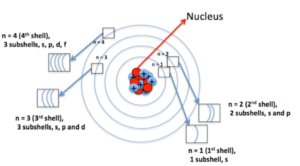Magnetic Quantum Number (m): It gives information about the orientation of orbitals in space. For a given ‘Ɩ’ value, there are 2Ɩ+1 possible values for m and these values are given by: m = – Ɩ to 0 to + lThus for Ɩ = 0, m = 0 {2(0)+1 = 1}. i.e. s sub shell contains only one orbital called s orbital.
For Ɩ = 1, mƖ = –1, 0 and +1, {2(1)+1 = 3}. i.e. p subshell contains three orbitals called p orbitals (px, py and pz).
For Ɩ = 2, m = –2, –1, 0, +1 and +2, {2(2)+1 = 5}. i.e. d subshell contains five orbitals called d orbitals (dxy, dyz, dzx, dx2-y2, dz2).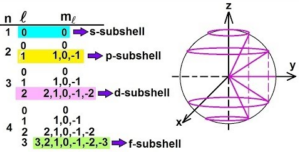Spin Quantum Number (s or ms): It refers to the orientation of the spin of the electron. It can have two values +1/2 and -1/2. +1/2 identifies the clockwise spin and -1/2 identifies the anti-clockwise spin.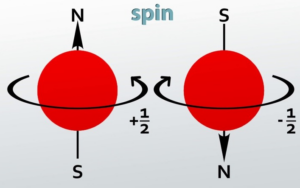Summary of Quantum Numbers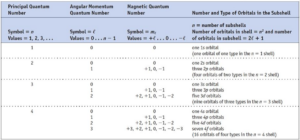Concept of Orbit and Orbital